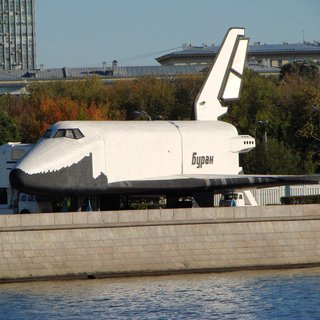OpenCV  4.0.0-alpha Open Source Computer Vision

## Introduction

In this tutorial you will learn how to use opencv_dnn module for image classification by using GoogLeNet trained network from Caffe model zoo.

We will demonstrate results of this example on the following picture.Buran space shuttle

## Source Code

We will be using snippets from the example application, that can be downloaded here.

#include <fstream>
#include <sstream>
#include <opencv2/dnn.hpp>
const char* keys =
"{ help h | | Print help message. }"
"{ input i | | Path to input image or video file. Skip this argument to capture frames from a camera.}"
"{ model m | | Path to a binary file of model contains trained weights. "
"It could be a file with extensions .caffemodel (Caffe), "
".pb (TensorFlow), .t7 or .net (Torch), .weights (Darknet) }"
"{ config c | | Path to a text file of model contains network configuration. "
"It could be a file with extensions .prototxt (Caffe), .pbtxt (TensorFlow), .cfg (Darknet) }"
"{ framework f | | Optional name of an origin framework of the model. Detect it automatically if it does not set. }"
"{ classes | | Optional path to a text file with names of classes. }"
"{ mean | | Preprocess input image by subtracting mean values. Mean values should be in BGR order and delimited by spaces. }"
"{ scale | 1 | Preprocess input image by multiplying on a scale factor. }"
"{ width | | Preprocess input image by resizing to a specific width. }"
"{ height | | Preprocess input image by resizing to a specific height. }"
"{ rgb | | Indicate that model works with RGB input images instead BGR ones. }"
"{ backend | 0 | Choose one of computation backends: "
"0: automatically (by default), "
"1: Halide language (http://halide-lang.org/), "
"2: Intel's Deep Learning Inference Engine (https://software.intel.com/openvino-toolkit), "
"3: OpenCV implementation }"
"{ target | 0 | Choose one of target computation devices: "
"0: CPU target (by default), "
"1: OpenCL, "
"2: OpenCL fp16 (half-float precision), "
"3: VPU }";
using namespace cv;
using namespace dnn;
std::vector<std::string> classes;
int main(int argc, char** argv)
{
CommandLineParser parser(argc, argv, keys);
parser.about("Use this script to run classification deep learning networks using OpenCV.");
if (argc == 1 || parser.has("help"))
{
parser.printMessage();
return 0;
}
float scale = parser.get<float>("scale");
Scalar mean = parser.get<Scalar>("mean");
bool swapRB = parser.get<bool>("rgb");
int inpWidth = parser.get<int>("width");
int inpHeight = parser.get<int>("height");
String model = parser.get<String>("model");
String config = parser.get<String>("config");
String framework = parser.get<String>("framework");
int backendId = parser.get<int>("backend");
int targetId = parser.get<int>("target");
// Open file with classes names.
if (parser.has("classes"))
{
std::string file = parser.get<String>("classes");
std::ifstream ifs(file.c_str());
if (!ifs.is_open())
std::string line;
while (std::getline(ifs, line))
{
classes.push_back(line);
}
}
if (!parser.check())
{
parser.printErrors();
return 1;
}
CV_Assert(!model.empty());
Net net = readNet(model, config, framework);
net.setPreferableBackend(backendId);
net.setPreferableTarget(targetId);
// Create a window
static const std::string kWinName = "Deep learning image classification in OpenCV";
if (parser.has("input"))
cap.open(parser.get<String>("input"));
else
cap.open(0);
// Process frames.
Mat frame, blob;
while (waitKey(1) < 0)
{
cap >> frame;
if (frame.empty())
{
break;
}
blobFromImage(frame, blob, scale, Size(inpWidth, inpHeight), mean, swapRB, false);
net.setInput(blob);
Mat prob = net.forward();
Point classIdPoint;
double confidence;
minMaxLoc(prob.reshape(1, 1), 0, &confidence, 0, &classIdPoint);
int classId = classIdPoint.x;
// Put efficiency information.
std::vector<double> layersTimes;
double freq = getTickFrequency() / 1000;
double t = net.getPerfProfile(layersTimes) / freq;
std::string label = format("Inference time: %.2f ms", t);
putText(frame, label, Point(0, 15), FONT_HERSHEY_SIMPLEX, 0.5, Scalar(0, 255, 0));
// Print predicted class.
label = format("%s: %.4f", (classes.empty() ? format("Class #%d", classId).c_str() :
classes[classId].c_str()),
confidence);
putText(frame, label, Point(0, 40), FONT_HERSHEY_SIMPLEX, 0.5, Scalar(0, 255, 0));
imshow(kWinName, frame);
}
return 0;
}

## Explanation

Also you need file with names of ILSVRC2012 classes: classification_classes_ILSVRC2012.txt.

Put these files into working dir of this program example.

2. Read and initialize network using path to .prototxt and .caffemodel files
Net net = readNet(model, config, framework);
net.setPreferableBackend(backendId);
net.setPreferableTarget(targetId);
You can skip an argument framework if one of the files model or config has an extension .caffemodel or .prototxt. This way function cv::dnn::readNet can automatically detects a model's format.
3. Read input image and convert to the blob, acceptable by GoogleNet

VideoCapture cap;
if (parser.has("input"))
cap.open(parser.get<String>("input"));
else
cap.open(0);

cv::VideoCapture can load both images and videos.

blobFromImage(frame, blob, scale, Size(inpWidth, inpHeight), mean, swapRB, false);

We convert the image to a 4-dimensional blob (so-called batch) with 1x3x224x224 shape after applying necessary pre-processing like resizing and mean subtraction (-104, -117, -123) for each blue, green and red channels correspondingly using cv::dnn::blobFromImage function.

4. Pass the blob to the network
net.setInput(blob);
5. Make forward pass
Mat prob = net.forward();
During the forward pass output of each network layer is computed, but in this example we need output from the last layer only.
6. Determine the best class
Point classIdPoint;
double confidence;
minMaxLoc(prob.reshape(1, 1), 0, &confidence, 0, &classIdPoint);
int classId = classIdPoint.x;
We put the output of network, which contain probabilities for each of 1000 ILSVRC2012 image classes, to the prob blob. And find the index of element with maximal value in this one. This index corresponds to the class of the image.
7. Run an example from command line
For our image we get prediction of class space shuttle with more than 99% sureness.